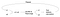# Stat tests when you don’t know what the distribution is- Permutation/Randomization Tests

Let’s first start with the use of Statistical tests with the help of an example-

A company called ‘pineapple’ wants to know whether there is an increase in sale if they give out a 10% discount on their app compared to the control group which is not given out any discount in the next three weeks, its necessary to account for a time duration because there might be users who are very active and otherwise.

In the above case, there are two conditions

1. Giving out a 10% discount
2. No discounts

Our null hypothesis is always the one in which we consider the status quo that is there are is no difference between the two conditions.

Ho: μ 1= μ2

Whereas the alternative would be

Ha: μ1 ≠ μ2

Stat test like T-tests, Z-tests assume the distribution of the data collected through the experiment to be normal and proceed on that to reject the null hypothesis or fail to reject the null hypothesis based on statistical significance but this is not always the case. That is where the Permutation/Randomization test comes in.

The motivation is that if the null hypothesis is true then any random arrangements of the data in the two groups would have the same pattern. That is if the samples for the two conditions are drawn from the same population then a shuffle and rearrangement of them would still give the same distribution in both conditions. For the example mentioned above if the mean sale in the discount group is \$53 and the control group is \$48 then the absolute difference of approx \$53–\$48=\$5 is observed. But to know whether it is statistically significant we would do the shuffling and rearranging and observe the probability of getting the mean difference of \$5 or higher and accordingly reject our null hypothesis or otherwise.

With n1 observations in condition 1 and n2 observations in condition 2, they can be shuffled together and rearranged by choosing n1/n2 out of n1+n2 to put in one condition. Then the rearranged values are averaged in both conditions and the p-value is compared to the significance. The motivation is if the null hypothesis is true then repeated random rearrangements of the values into two groups would not produce a statistically significant difference between the means.

In the permutation test, all kind of rearrangements is carried out that is

Number of permutations=n1+n2 choose n1= n1+n2 choose n2

which is computationally expensive therefore the randomization test is preferred where resampling and test statistic is repeated in the order of 1000–10000. After which the usual steps such as p-value comparison is carried out.Permutation/randomization test

Permutation/Randomization tests are free from the assumption of Normalization which makes them a lot more robust compared to more common test statistics.

Machine Learning Engineer at Yaar| Data Science | ML| Statistics

## More from Apurva Misra

Machine Learning Engineer at Yaar| Data Science | ML| Statistics